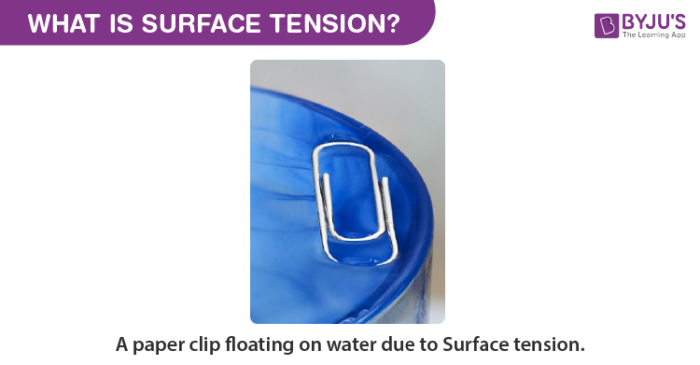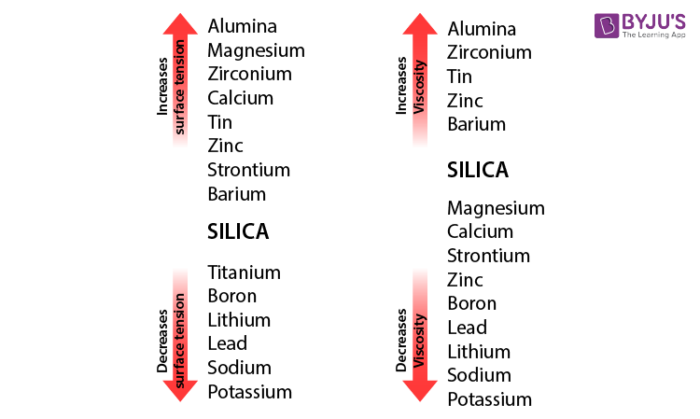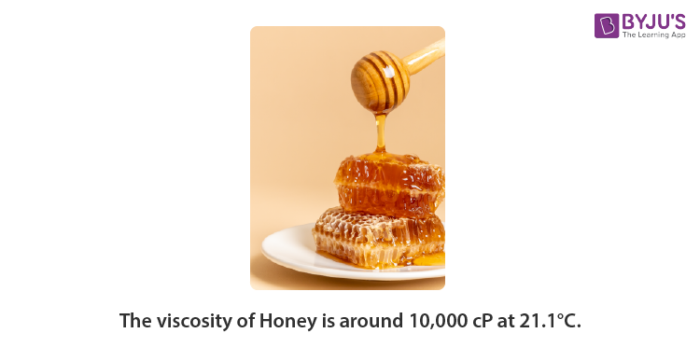Checkout JEE MAINS 2022 Question Paper Analysis : Checkout JEE MAINS 2022 Question Paper Analysis :

# Viscosity And Surface Tension

Viscosity and surface tension, are dependent on molecular interactions.

• However, viscosity results due to collaboration among molecules of the identical molecules located in the same material (in case of fluids).
• Whereas surface tension is determined by the difference of interactions between the molecules of the material (fluid) with the molecules of the material in contact.

## What is Surface Tension?

Surface tension is the amount of energy required to increase the surface of the liquid by unit area. In other words, it is also the property of the liquid surface that resists force. Intuitively, it keeps a barrier between foreign materials and liquid as well as this is the force that holds the liquid molecules bind together.For example, if we add soap to the water, it is surface tension decreases, thereby the liquid with soap easily mixes with dirt on hand and thus cleans it. It is also the property of the liquid surface that resists force.Intuitively, it keeps a barrier between foreign materials and the liquid. This is the force that holds the liquid molecules bonded together.

If we add soap to the water, it is surface tension decreases, thereby the liquid with soap easily mixes with dirt on hand and thus cleans it.

Surface tension is sometimes seen in terms of energy. That is more is the surface tension, more is the energy and thus to minimize energy the fluids assume the shape with the smallest surface area. This is the reason behind the water droplets being spherical. A sphere has a minimal surface area, for a given volume.

## What is Viscosity?

Viscosity is the resistance of a fluid to flow.For example, oil has a high viscosity, but when we put it in the car and heat it up, it becomes less viscous and thus flows smoothly through an engine and other parts of the car. Higher the viscosity, slow will be the liquid flow. Viscosity decreases with increase in temperature, with some exceptions.

Viscosity is sometimes also understood as frictional forces that act in between fluid and the surface of contact. The surface can either be a solid surface like a pipe and water can be the fluid. Now the resistance provided by the pipe to the water flow is viscosity. However, viscosity can also arise in between two fluids flowing at different velocities.

The above phenomenon of viscosity is very much particular to liquid, to be precise Newtonian fluids. However, in the case of non-Newtonian fluids, the interactions are quite bizarre and are always hard to model and understand.